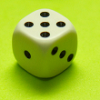#### You may also likeFour fair dice are marked differently on their six faces. Choose first ANY one of them. I can always choose another that will give me a better chance of winning. Investigate.### How Many Dice?

A standard die has the numbers 1, 2 and 3 are opposite 6, 5 and 4 respectively so that opposite faces add to 7? If you make standard dice by writing 1, 2, 3, 4, 5, 6 on blank cubes you will find there are 2 and only 2 different standard dice. Can you prove this ?### Dicing with Numbers

In how many ways can you arrange three dice side by side on a surface so that the sum of the numbers on each of the four faces (top, bottom, front and back) is equal?

# Troublesome Dice

### Why do this problem?

This problem offers students the chance to visualise in three dimensions (using the context of dice), list possibilities systematically, and work on probabilities in a less familiar context.

### Possible approach

Begin with some visualising of dice:
"Imagine a six-sided die, where the opposite faces add up to 7. Rotate your die so that the top face shows 1. What does your bottom face show?"
"Now rotate it round so that the front face shows 5. What will the back face show?"
"What numbers are on the other two faces? Think about what's on the left and right faces of your die. Are you all picturing the same die?"
These questions tease out an understanding that all 6-sided dice whose opposite faces sum to seven are essentially the same, the only possible difference being that you can make a "right-handed" and "left-handed" version that are mirror images of each other (read more here.)

The problem Right or Left? explores the differences between right- and left-handed dice.

Now introduce the context of the problem and set the class to working out all the possible edge scores and how these could be distributed so that everyone has an equal chance of getting the pie, when there are two, three, four... people sharing the meal.

After everyone has shared their work on this task, the same analysis can be done with the die that always lands "corner-up".

### Key questions

Numbers on opposite faces sum to 7. Does this imply that numbers NOT on opposite faces DO NOT sum to 7?
The smallest edge total is 3, and the largest is 11. How do I know that at least one of the numbers between 3 and 11 can be made in more than one way?
The smallest corner total is 6, and the largest is 15. How do I know that at least two of the numbers in between must be impossible to make?

### Possible extension

Discuss whether the probabilities can be combined, and whether it's equally likely that the die will land "face-up", "edge-up" or "corner-up" - these ideas are developed in the problem Dicey Decisions.

### Possible support

Offer students dice to use while working on the problem.